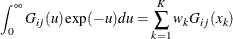# The MDC Procedure

### Heteroscedastic Extreme-Value Model

The heteroscedastic extreme-value (HEV) model (Bhat, 1995) allows the random components of the utility function to be nonidentical. Specifically, the HEV model assumes independent but nonidentical error terms distributed with the Type I extreme-value distribution. The HEV model allows the variances of the random components of utility to differ across alternatives. Bhat (1995) argues that the HEV model does not have the IIA property. The HEV model contains the conditional logit model as a special case. The probability that an individual i will choose alternative j from the set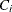of available alternatives is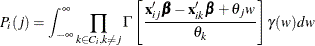where the choice sethas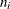elements and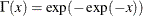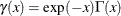are the cumulative distribution function and probability density function of the Type I extreme-value distribution. The variance of the error term for the jth alternative is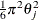. If the scale parameters,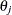, of the random components of utility of all alternatives are equal, then this choice probability is the same as that of the conditional logit model. The log-likelihood function of the HEV model can be written as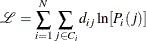where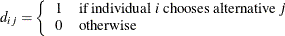Since the log-likelihood function contains an improper integral function, it is computationally difficult to get a stable estimate. With the transformation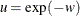, the probability can be written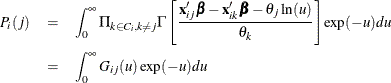Using the Gauss-Laguerre weight function,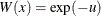, the integration of the log-likelihood function can be replaced with a summation as follows: